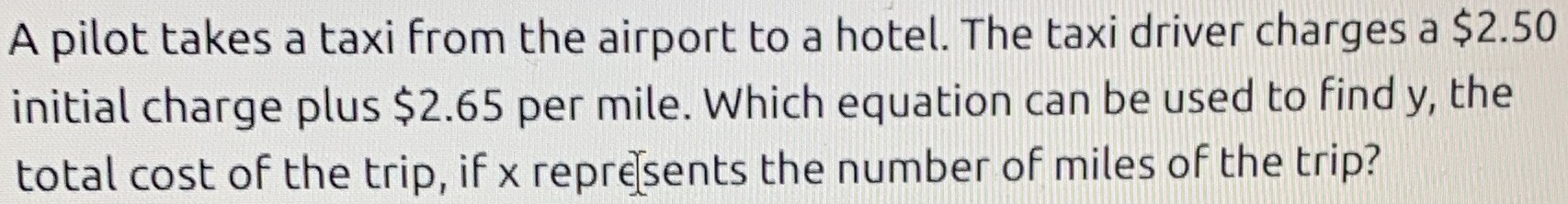### ¿Todavía tienes preguntas de matemáticas?

Pregunte a nuestros tutores expertos
Algebra
PreguntaA pilot takes a taxi from the airport to a hotel. The taxi driver charges a $$\ 2.50$$ initial charge plus $$\ 2.65$$ per mile. Which equation can be used to find $$y ,$$ the total cost of the trip, if $$x$$ reprejsents the number of miles of the trip?